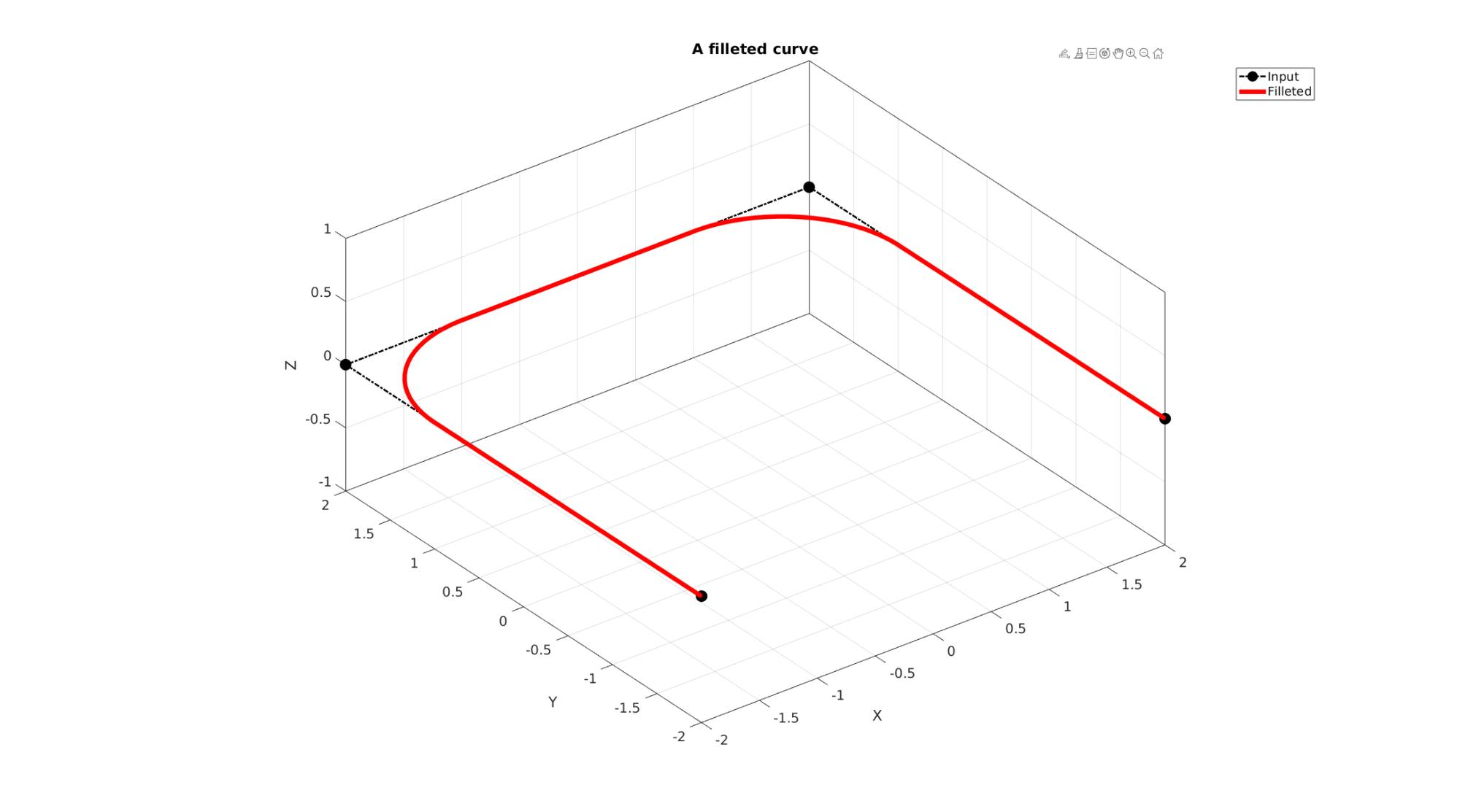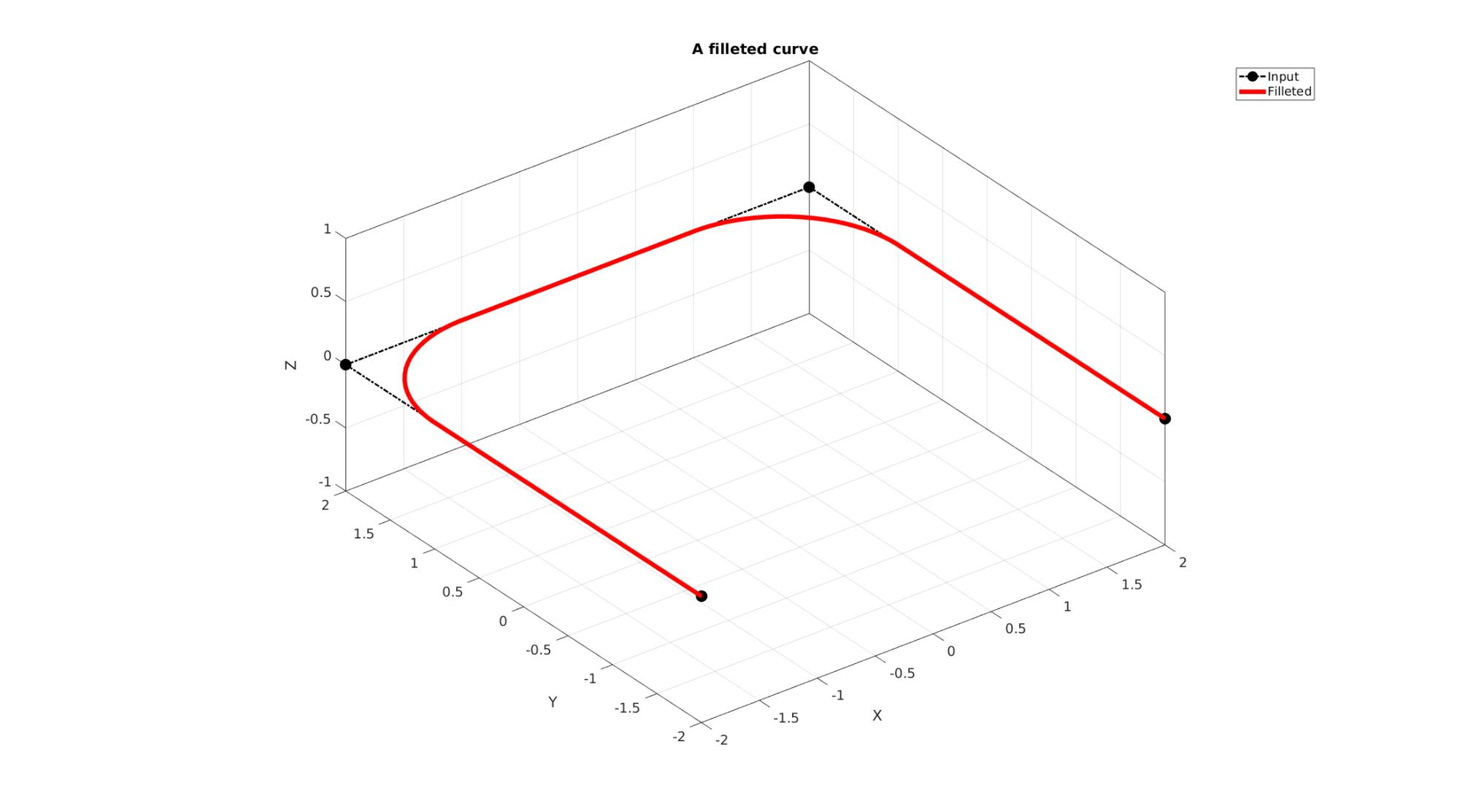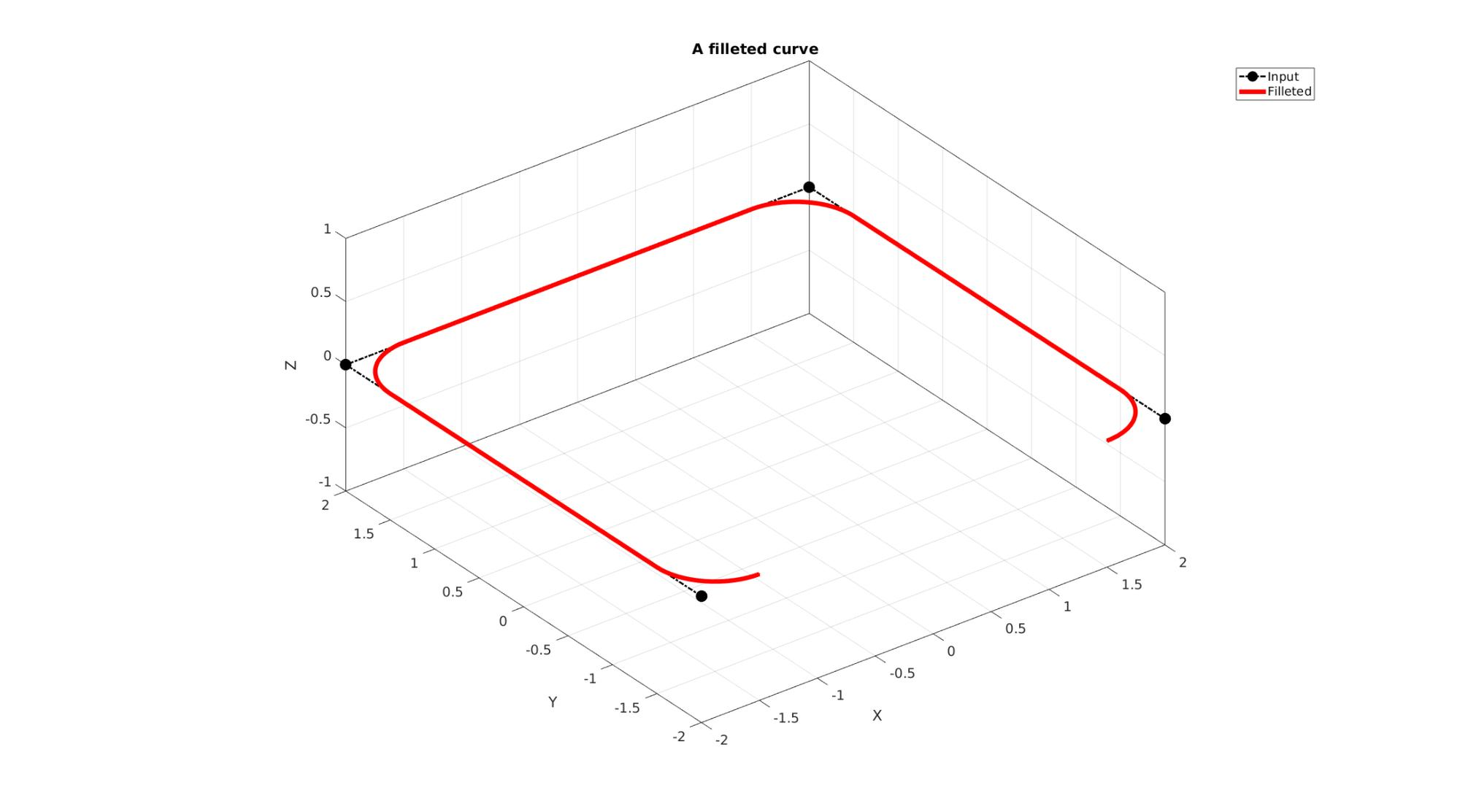# filletCurve

Below is a demonstration of the features of the filletCurve function

## Syntax

[VN]=filletCurve(Vt,r,np,closedLoopOption);

## Description

This function fillets a curve based on the input radius r using np points per fillet arc. If closedLoopOpt==1 then closed end conditions are used such that the end and start regions are also filleted.

## Examples

```clear; close all; clc;
```

Plot settings

```fontSize=15;
markerSize1=45;
lineWidth1=2;
lineWidth2=5;
lineWidth3=2;
faceAlpha=0.5;
```

## Example: Filleting a curve in 3D

Simulating a curve with sharp features

```Vt=[0 0 0; 10 0 0; 5 10 0; 10 0 10; 0 10 10; ];
```
```%Setting control parameters
np=25; %Number of points used to construct each fillet edge
closedLoopOption=0; %Use 1 if curve represents a closed loop but containes unique points
[VN]=filletCurve(Vt,r,np,closedLoopOption);
```

Plotting results

```hf1=cFigure;
title('A filleted curve','FontSize',fontSize);
xlabel('X','FontSize',fontSize);ylabel('Y','FontSize',fontSize); zlabel('Z','FontSize',fontSize);
hold on;

plotV(Vt,'k.-.','lineWidth',lineWidth1,'MarkerSize',markerSize1);
plotV(VN,'r.-','lineWidth',lineWidth2);

axis equal; view(3); axis tight;  grid on;  set(gca,'FontSize',fontSize);
drawnow;
```## Example: Filleting a closed curve in 3D

```closedLoopOption=1;
[VN]=filletCurve(Vt,r,np,closedLoopOption);
```

Plotting results

```hf2=cFigure;
title('A filleted curve based on closed end conditions','FontSize',fontSize);
xlabel('X','FontSize',fontSize);ylabel('Y','FontSize',fontSize); zlabel('Z','FontSize',fontSize);
hold on;

plotV(Vt,'k.-.','lineWidth',lineWidth1,'MarkerSize',markerSize1);
plotV([Vt(1,:);Vt(end,:)],'g.-.','lineWidth',lineWidth1,'MarkerSize',markerSize1);
plotV(VN,'r.-','lineWidth',lineWidth2);

axis equal; view(3); axis tight;  grid on;  set(gca,'FontSize',fontSize);
drawnow;
```## Example: Extruding a filleted curve for CAD like model building

```%Sketching side profile
x=[0 0 5 15 15];
y=[0 4 9 10 0];
V=10*[x(:) y(:)];

%Fillet sketch
np=50; %Number of points used to construct each fillet edge
closedLoopOption=0; %Use 1 if curve represents a closed loop but containes unique points
[Vc]=filletCurve(V,r,np,closedLoopOption);
```

Plotting sketch

```hf1=cFigure;
title('The side profile sketch','FontSize',fontSize);
xlabel('X','FontSize',fontSize);ylabel('Y','FontSize',fontSize); zlabel('Z','FontSize',fontSize);
hold on;

plotV(V,'k.-.','lineWidth',lineWidth1,'MarkerSize',markerSize1);
plotV(Vc,'r-','lineWidth',lineWidth2,'MarkerSize',markerSize1);

axis equal; view(2); axis tight;  grid on;  set(gca,'FontSize',fontSize);
drawnow;
```Extruding model

```cPar.pointSpacing=10;
cPar.depth=85;
cPar.patchType='tri';
cPar.dir=0;
cPar.n=[0 0 1];
cPar.closeLoopOpt=0;

[F_tri,V_tri]=polyExtrude(Vc,cPar);
```

Plotting meshed model

```hf2=cFigure;
title('The extruded model mesh','FontSize',fontSize);
xlabel('X','FontSize',fontSize);ylabel('Y','FontSize',fontSize); zlabel('Z','FontSize',fontSize);
hold on;

hp=patch('faces',F_tri,'Vertices',V_tri);

set(hp,'FaceColor','g','EdgeColor','k','FaceAlpha',1);
axis equal; view(3); axis tight;  grid on;  set(gca,'FontSize',fontSize);
drawnow;
```GIBBON www.gibboncode.org

Kevin Mattheus Moerman, [email protected]

GIBBON footer text

GIBBON: The Geometry and Image-based Bioengineering add-On. A toolbox for image segmentation, image-based modeling, meshing, and finite element analysis.

Copyright (C) 2019 Kevin Mattheus Moerman

This program is free software: you can redistribute it and/or modify it under the terms of the GNU General Public License as published by the Free Software Foundation, either version 3 of the License, or (at your option) any later version.

This program is distributed in the hope that it will be useful, but WITHOUT ANY WARRANTY; without even the implied warranty of MERCHANTABILITY or FITNESS FOR A PARTICULAR PURPOSE. See the GNU General Public License for more details.

You should have received a copy of the GNU General Public License along with this program. If not, see http://www.gnu.org/licenses/.